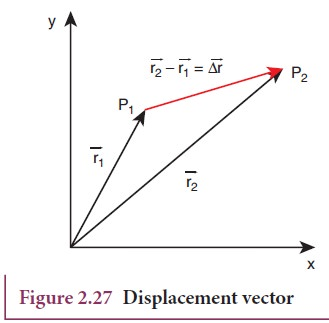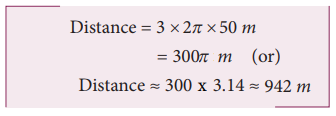Home | | Physics 11th std | Distance and Displacement

# Distance and Displacement

Distance is the actual path length travelled by an object in the given interval of time during the motion.

DISTANCE AND DISPLACEMENT

Distance is the actual path length travelled by an object in the given interval of time during the motion. It is a positive scalar quantity.

Displacement is the difference between the final and initial positions of the object in a given interval of time. It can also be defined as the shortest distance between these two positions of the object and its direction is from the initial to final position of the object, during the given interval of time. It is a vector quantity. Figure 2.26 illustrates the difference between displacement and distance.## Displacement Vector in Cartesian Coordinate System

In terms of position vector, the displacement vector is given as follows. Let us consider a particle moving from a point P1 having position vectorThis displacement is also shown in Figure 2.27.## Solved Example Problems for Distance and Displacement

### Example 2.15

Assume your school is located 2 km away from your home. In the morning you are going to school and in the evening you come back home. In this entire trip what is the distance travelled and the displacement covered?

### SolutionThe displacement covered is zero. It is because your initial and final positions are the same.

But the distance travelled is 4 km.

### Example 2.16

An athlete covers 3 rounds on a circular track of radius 50 m. Calculate the total distance and displacement travelled by him.

### SolutionThe total distance the athlete covered =3x circumference of trackThe displacement is zero, since the athlete reaches the same point A after three rounds from where he started.

## Solved Example Problems for Displacement Vector in Cartesian Coordinate System

### Example 2.17

Calculate the displacement vector for a particle moving from a point P to Q as shown below. Calculate the magnitude of displacement.### SolutionTags : Cartesian Coordinate System, Solved Example Problems , 11th Physics : UNIT 2 : Kinematics
Study Material, Lecturing Notes, Assignment, Reference, Wiki description explanation, brief detail
11th Physics : UNIT 2 : Kinematics : Distance and Displacement | Cartesian Coordinate System, Solved Example Problems